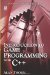# 9.1 Functions in Mathematics

## 9.1 Functions in Mathematics

In mathematics, when one quantity depends on a second quantity, the first is said to be a function of the second. The calculation of the area of a circle, for example, depends on the radius. So, the area of a circle is a function of the radius. Consider the following equation:

• y = 3x 5

This equation can be considered a function in which x is the independent variable and y is the dependent variable. In terms of a function, x is the domain of the function, and can be any number. The entire scope of numbers x can assume is called the range, such as 1, 2, 3, and so on. y is said to be the value of the function. This makes sense because, for whatever number x can be, there will also be a corresponding value for y.

### 9.1.1 Function Notation

In mathematics there is a notation reserved for representing functions. Consider the following two functions:

 y = 5x + 3 y = 9x − 7

These are two separate functions, and each can be given a name. Since f is often used to denote a function, we'll call the first one f and the second one g.

Here are the two functions f and g again. The parentheses are used after the name of the function and inside the parentheses are the arguments of the function.

 f(x) = 5x + 3 g(x) = 9x − 7

These functions have one argument each, namely x. So, each function expects a value for x as its input. Consider the following:

f(x) = 5x + 3

Where x = 3:

f(3) = 15 + 3 = 18

Since functions evaluate to a single value, functions can also be used as arguments to other functions. This is demonstrated in the following example. g is evaluated first and its value is passed on to f as an argument.

• f(g(x))Introduction to Game Programming with C++ (Wordware Game Developers Library)
ISBN: 1598220322
EAN: 2147483647
Year: 2007
Pages: 225
Authors: Alan Thorn

Similar book on Amazon

flylib.com © 2008-2017.
If you may any questions please contact us: flylib@qtcs.net